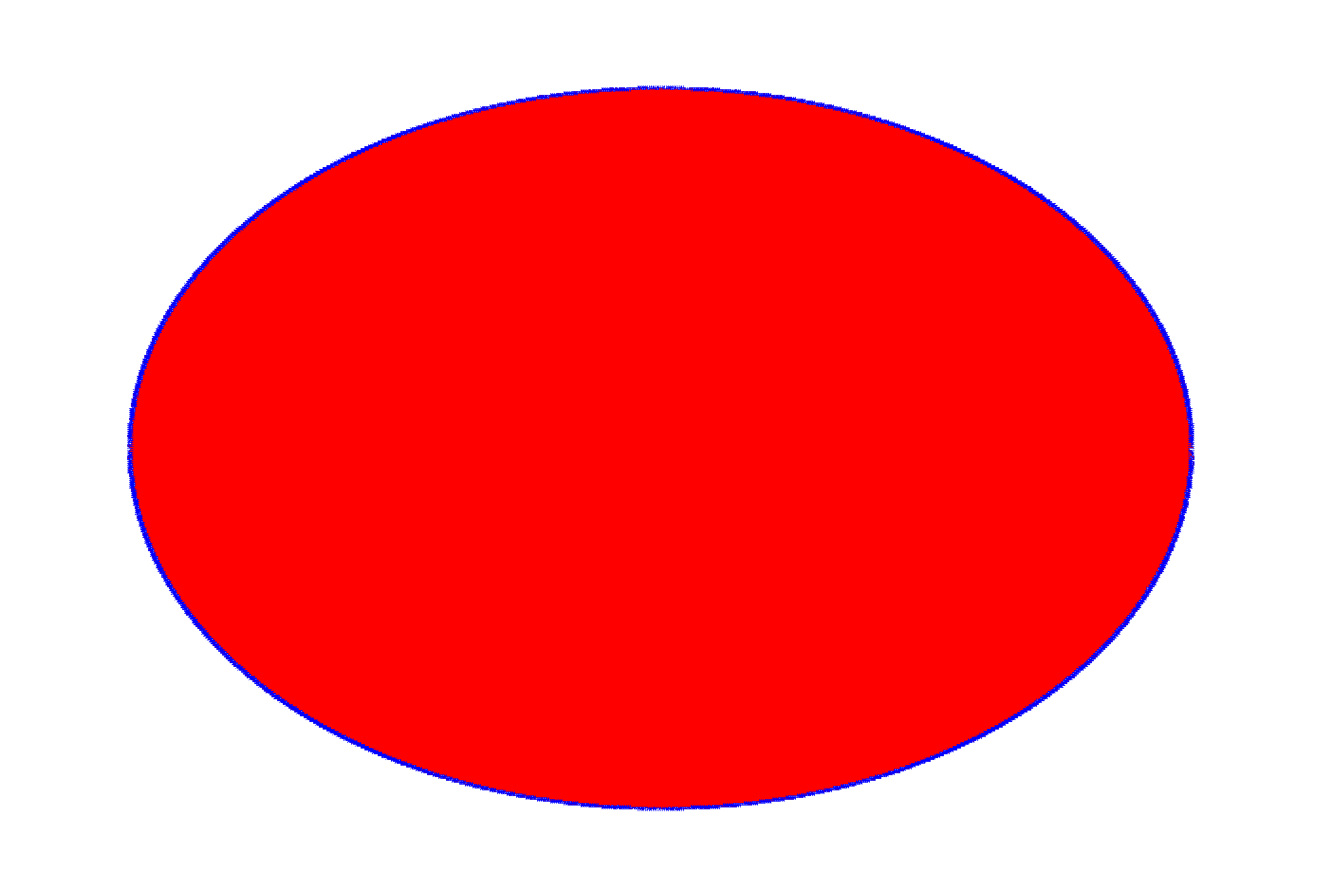ROOT   Reference Guidepolytest1.C File Reference

## Detailed DescriptionThis macro is testing the "compacting" algorithm in TPadPainter. It reduces the number of polygon's vertices using actual pixel coordinates.It's not really useful, but just to test that the resulting polygon is still reasonable. Initial number of points is 1000000, after "compression" it's 523904 (with default canvas size, before you tried to resize it) - so almost half of vertices were removed but you can still see the reasonable shape. If you resize a canvas to a smaller size, the number of vertices after compression can be something like 5000 and even less. It's easy to 'fool' this algorithm though in this particular case (ellipse is a kind of fringe case, you can easily have a sequence of almost unique vertices (at a pixel level).

//Includes for ACLiC.
#include <cassert>
#include <vector>
#include "TRandom.h"
#include "TCanvas.h"
#include "TError.h"
#include "Rtypes.h"
#include "TNamed.h"
#include "TMath.h"
class PolyTest1 : public TNamed, public TAttLine, public TAttFill {
public:
PolyTest1(unsigned nVertices);
void Paint(const Option_t *notUsed);
void Reset(unsigned nVertices);
private:
enum {
kNPointsDefault = 10000//minimal number of points.
};
std::vector<Double_t> fXs;
std::vector<Double_t> fYs;
};
//_____________________________________________________________
PolyTest1::PolyTest1(unsigned nVertices)
: TNamed("polygon_compression_test1", "polygon_compression_test1")
{
Reset(nVertices);
}
//_____________________________________________________________
void PolyTest1::Reset(unsigned nVertices)
{
//Some canvas must already exist by this point.
//We need a gRandom to exist.
assert(gRandom != 0 && "Reset, gRandom is null");
if (nVertices < kNPointsDefault) {
Warning("Reset", "resetting nVertices parameter to %u", unsigned(kNPointsDefault));
nVertices = kNPointsDefault;
}
fXs.resize(nVertices);
fYs.resize(nVertices);
Double_t xMin = 0., xMax = 0., yMin = 0., yMax = 0.;
assert(xMax - xMin > 0 && yMax - yMin > 0 && "Reset, invalid canvas' ranges");
const Double_t xCentre = xMin + 0.5 * (xMax - xMin);
const Double_t yCentre = yMin + 0.5 * (yMax - yMin);
const Double_t r = TMath::Min(xMax - xMin, yMax - yMin) * 0.8 / 2;
const Double_t angle = TMath::TwoPi() / (nVertices - 1);
for (unsigned i = 0; i < nVertices - 1; ++i) {
const Double_t currR = r + gRandom->Rndm() * r * 0.01;
fXs[i] = xCentre + currR * TMath::Cos(angle * i);
fYs[i] = yCentre + currR * TMath::Sin(angle * i);
}
fXs[nVertices - 1] = fXs;
fYs[nVertices - 1] = fYs;
}
//_____________________________________________________________
void PolyTest1::Paint(const Option_t * /*notUsed*/)
{
}
void polytest1()
{
TCanvas * const cnv = new TCanvas;
cnv->cd();
PolyTest1 * polygon = new PolyTest1(1000000);
polygon->SetLineColor(kBlue);
polygon->SetFillColor(kRed);
polygon->SetLineWidth(1);
polygon->Draw();//Attach a polygon to a canvas.
}

Definition in file polytest1.C.

Warning
void Warning(const char *location, const char *msgfmt,...)
Use this function in warning situations.
Definition: TError.cxx:232
TAttFill::Modify
virtual void Modify()
Change current fill area attributes if necessary.
Definition: TAttFill.cxx:211
TMath::Cos
Double_t Cos(Double_t)
Definition: TMath.h:630
r
ROOT::R::TRInterface & r
Definition: Object.C:4
TNamed.h
TRandom.h
Int_t
int Int_t
Definition: RtypesCore.h:45
TCanvas.h
TAttLine::Modify
virtual void Modify()
Change current line attributes if necessary.
Definition: TAttLine.cxx:242
TObject::Paint
virtual void Paint(Option_t *option="")
This method must be overridden if a class wants to paint itself.
Definition: TObject.cxx:521
Option_t
const typedef char Option_t
Definition: RtypesCore.h:66
TAttLine
Definition: TAttLine.h:18
TCanvas::cd
Definition: TCanvas.cxx:704
TNamed
Definition: TNamed.h:29
gRandom
R__EXTERN TRandom * gRandom
Definition: TRandom.h:62
TMath::Sin
Double_t Sin(Double_t)
Definition: TMath.h:626
TRandom::Rndm
virtual Double_t Rndm()
Machine independent random number generator.
Definition: TRandom.cxx:541
kRed
@ kRed
Definition: Rtypes.h:66
TMath::Min
Short_t Min(Short_t a, Short_t b)
Definition: TMathBase.h:180
TMath::TwoPi
constexpr Double_t TwoPi()
Definition: TMath.h:50
Double_t
double Double_t
Definition: RtypesCore.h:59
TCanvas
Definition: TCanvas.h:23
kBlue
@ kBlue
Definition: Rtypes.h:66